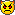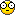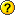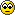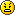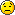Project
 Navigation Home Contact GuestbookSome astounding science facts Some astounding maths facts Newsletter Counter Ways to Achieve GodSome astounding maths facts

There are many questions on how things works, how they occour....
Here, some of them would be solved.

1. Prove 1=2

A. Suppose a=b

So, ab=b2
SO, ab-a2=b2-a2
SO, a(b-a)=(b-a)(b+a)

So, a(b-a)=(b+a)(b-a)
So, a=b+a
But, a=b
So, b=a
So, a=a+a
So, a=2a
So, 1=2
Hence proved.

2. To find 'n' in the arithmatic progression(A.P.)
Aryabhatta-1 gave the formula to find the value of  number of term in A.P. But, I shortened it to:
((l - a)/d)+1
where, l = given last term, a= first term and d= difference between two terms.
If, a and d are same then 'n' can be found by :
l/d
where, l= given last term and d= difference between two terms.

3. To prove  0=any number.
A. Suppose a=b

So, ab=b2
SO, ab-a2=b2-a2
SO, a(b-a)=(b-a)(b+a)

So, a(b-a)=(b+a)(b-a)
So, a=b+a
But, a=b
So, b=a
So, a=a+a
So, a=2a
So, 0=2a-a
So, 0=a.
Here, a can be any number. So, 0=any number is proved to be true.

4. 3=2

Can any one prove 3=2. I hope none. But Ramanujam had.

See this illustration:

-6 = -6

9-15 = 4-10

Adding 25/4 to both sides:

9-15+(25/4) = 4-10+(25/4 )

Changing the order

9+(25/4)-15 = 4+(25/4)-10

[This is just like : a square + b square - two a b = (a-b)square.]

Here a = 3, b=5/2 for L.H.S....... and a =2, b=5/2 for R.H.S.

So it can be expressed as follows

(3-5/2)(3-5/2) = (2-5/2)(2-5/2)

Taking positive square root on both sides.

3 - 5/2 = 2 - 5/2

3 = 2

5. To prove 1=any number
Let 'a' be any number.
So, a * 0 = 0.
So, a = 0 / 0.
But, value of '0' is not known. So, let value of '0' be 'x'.
So, a = x / x
So, a=1.
Hence proved.

6. To prove 1 = 0.
Now, 0 = any number (see proof no. 3)
and, 1= any number (see proof no. 5)
So, 1 = 0.
Hence proved.

7. To prove negative integer = positive integer.
0 = any number (see proof no. 3)
So, 0 = a                                  (Here, 'a' is short form of 'any
number')
So,  (-a) = 0.                    (Tanking 'a' on other side)
Also, 0 = 0.
So, (-a) = 0 = a.
So, (-a) = a.
Hence, negative integer =positive integer.
Hence proved.

Can u find any flaws??

how was that??cool isn't it!!